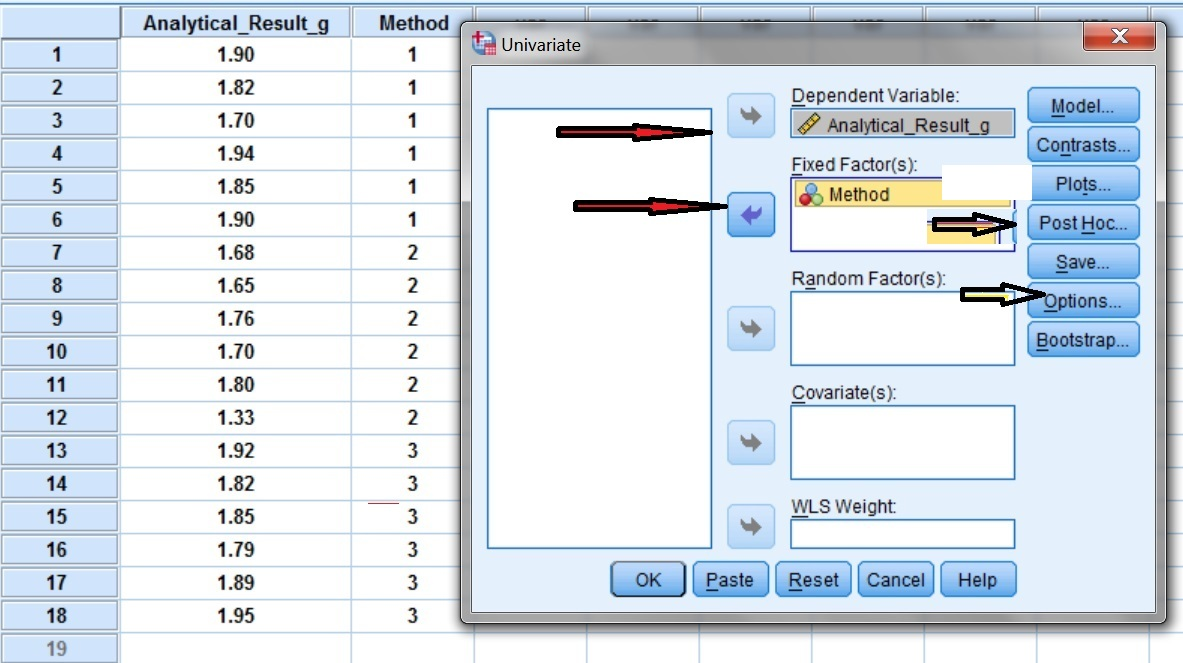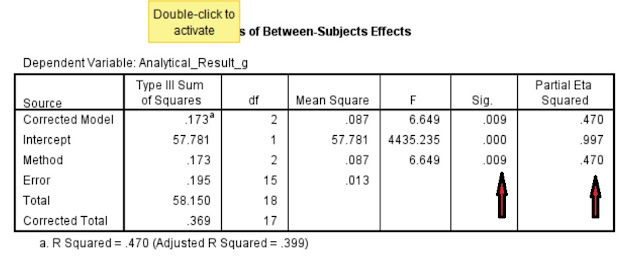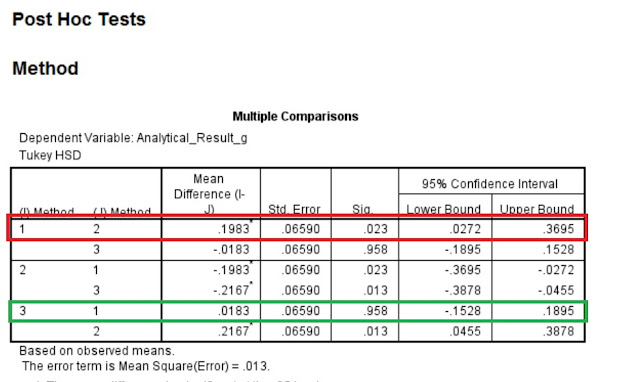Comparing several group means by one-way ANOVA - Post Hoc tests using SPSS | Chemistry Net

# Comparing several group means by one-way ANOVA - Post Hoc tests using SPSS

Comparing several Group Means by ANOVA using SPSS

# Comparing of several Group Means by One-Way ANOVA using SPSS - Post Hoc Tests

In a previous post entitled "Comparing several Group Means by One-Way Anova using SPSS" the one-way ANOVA test was presented. An example was given where the situation encountered was to compare mean results for the concentration of an analyte obtained by threel different methods. The dependent variable was the mean analytical results (labeled Analytical_Result_g) while the independent variable was the method used (labeled Method)

It is common in analytical work to run experiments in which there are three, four or even five levels of the independent variable (that can cause variation of the results in addition to random error of measurements) and in these cases the technique called analysis of variance (ANOVA) is used. ANOVA is an extremely powerful statistical technique for analysis of data that has the advantage that it can be used to analyze situations in which there are several independent variables (or better several levels of the independent variable).

The output of the ANOVA test showed that the mean results of the concentration of the analyte by the three methods used were not equal. In this post we are going to answer which mean differs from which mean by using Post Hoc tests. Post hoc tests (also called post hoc comparisons, multiple comparison tests, follow-up tests) are tests of the statistical significance of differences between group means calculated after - "post"- having done an analysis of variance (ANOVA) that shows an overall difference. The F ratio of the ANOVA indicates that some sort of statistically significant differences exist somewhere among the groups being studied. Post hoc analyses are meant to specify what kind and where.

There are various Post Hoc tests such as: Tukey's Honestly Significant Difference (HSD) test, Scheffe test, Newman-Keuls test and Duncan's Multiple Range test. If the assumption of homogeinity of variance has been met (equal variances assummed) - in our case has been proven in our previous post entitled "Comparing several Group Means by One-Way Anova using SPSS" - Tukey's test is used.

There are many ways to run the exact same ANOVA in SPSS. This time the General Linear Model is going to be used because it will provide us with an estimate for the effect size of our model (labeled as partial eta squared). The effect size will show us what percentage of the variance of the analytical results (of the dependent variable) can be accounted to the different methods used (of the independent variable).

Let us use again the same Example I.1

Example I.1

Figure I.1 shows the analytical results obtained regarding the weight of Au (in grams/ton) in a certified reference material X. Three different methods (Method 1, 2 and 3) were used for the determination and six replicate measurements were made in each case. Is there a significant difference in the means calculated by each method?In SPSS access the main dialog box using Analyze --------> General Linear Model --------> Univariate (Fig. I.1)In SPSS select as Dependent Variable: Analytical_Result_g and as Fixed Factor (independent variable): Method (Fig. I.2). Select also Post Hoc and Options tests.The selection Post Hoc is pressed (Fig. I.3) and the independent variable method is selected for the Post Hoc tests (Fig. I.3). Then select the Tukey test and click Continue.The selection Options is pressed (Fig. I.4) and the Estimates of the effect size is selected. Continue is pressed.The SPSS ANOVA output of Between Subjects Effects is shown in Fig. I.5. The mean results for the dependent variable Analytical_Result_g obtained by the 3 different methods differ significantly since the p value denoted by Sig = 0.009 < 0.05. The result for the p value - as expected -is exactly the same with that obtained in the previous post mentionned above. However, an estimate of the effect size is given by this ANOVA test labeled as Partial Eta Squared. The different methods used account for some 47% (given as .470) of the variance in the means of the Analytical_Result_g.From the results shown in the output of Between Subjects Effects (Fig. I.5) it appears that the 3 means compared differ significantly. But exactly which mean differs from which mean?

Certainly, histograms and the mean table that were given in the post "Comparing several Group Means by One-Way Anova using SPSS" gave us a clue. A more formal answer is given by the Tukey's test in the Multiple Comparisons table (Fig. I.6). Statistically significant mean differences are flagged with an asterisk (*). For instance, the very first line indicates that Method 1 has a mean value 0.2 higher than the mean value of Method 2 and this is statistically significant since Sig = 0.023 < 0.05. Also since the confidence interval is not including zero means that zero difference between these means is unlikely.Method 3 has a mean value 0.02 higher than the mean value of Method 1 and this is not statistically significant since Sig = 0.958 > 0.05. Also since the confidence interval is including zero means that zero difference between these means is likely.

References

1. D.B. Hibbert, J.J. Gooding, "Data Analysis for Chemistry", Oxford Univ. Press, 2005
2. J.C. Miller and J.N Miller, “Statistics for Analytical Chemistry”, Ellis Horwood Prentice Hall, 2008
3. Steven S. Zumdahl, “Chemical Principles” 6th Edition, Houghton Mifflin Company, 2009
4. D. Harvey, “Modern Analytical Chemistry”, McGraw-Hill Companies Inc., 2000
5. R.D. Brown, “Introduction to Chemical Analysis”, McGraw-Hill Companies Inc, 1982
6. S.L.R. Ellison, V.J. Barwick, T.J.D. Farrant, “Practical Statistics for the Analytical Scientist”, 2nd Edition, Royal Society of Chemistry, 2009
7. A. Field, “Discovering Statistics using SPSS” , Sage Publications Ltd., 2005

Key Terms

comparing several means, analysis of variance,post hoc tests, ANOVA, t-tests,